## DataRay Blog

February 26, 2016

# Flat-Top Beams and Plateau Uniformity Calculations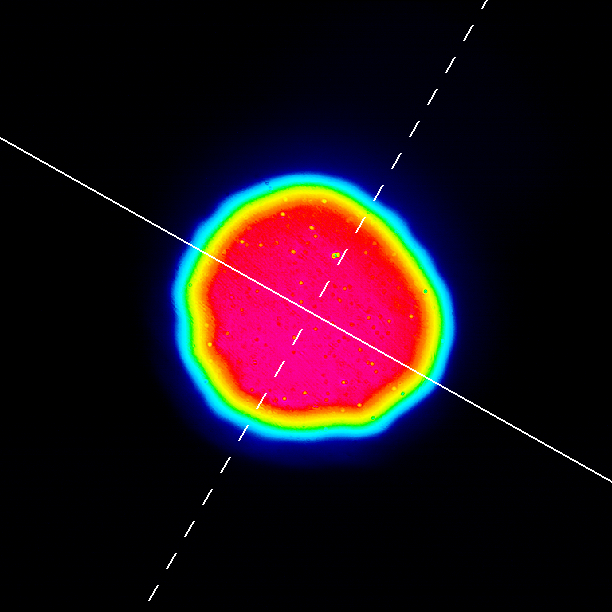Figure 1: Flat-top beam image.

A flat-top beam (otherwise known as a top-hat) is used in various applications where a controlled profile is desired (i.e. a uniform density across the irradiated surface). Passing a Gaussian beam through special optics will create the flat-top beam and is the most common way of generating a flat-top beam. Uniform excimer beams are used in many material processing applications (e.g. creating integrated circuits). Industries such as welding, drilling, and medicine also make extensive use of flat-top beams. Although a rough estimate of a flat-top beam’s uniformity can be gauged visually using the image of the beam profile taken with a CCD or CMOS camera, a more accurate method is needed. The DataRay software (versions LCMvD23 and later) reports the plateau uniformity value, which gives the full width half maximum (FWHM) width of the beam’s power density histogram. The plateau uniformity of the flat-top beam is a value between zero and one used to quantify the uniformity of the flat-top beam. Zero represents a perfect flat-top beam. As the flat-top beam deviates from the theoretical flat-top, the value moves towards one. DataRay adheres to the ISO 13694 procedure for calculating the plateau uniformity. The plateau uniformity has the advantage of providing a single metric to characterize the beam uniformity across the entirety of the beam profile, rather than looking at individual slices of the beam.

#### Histogram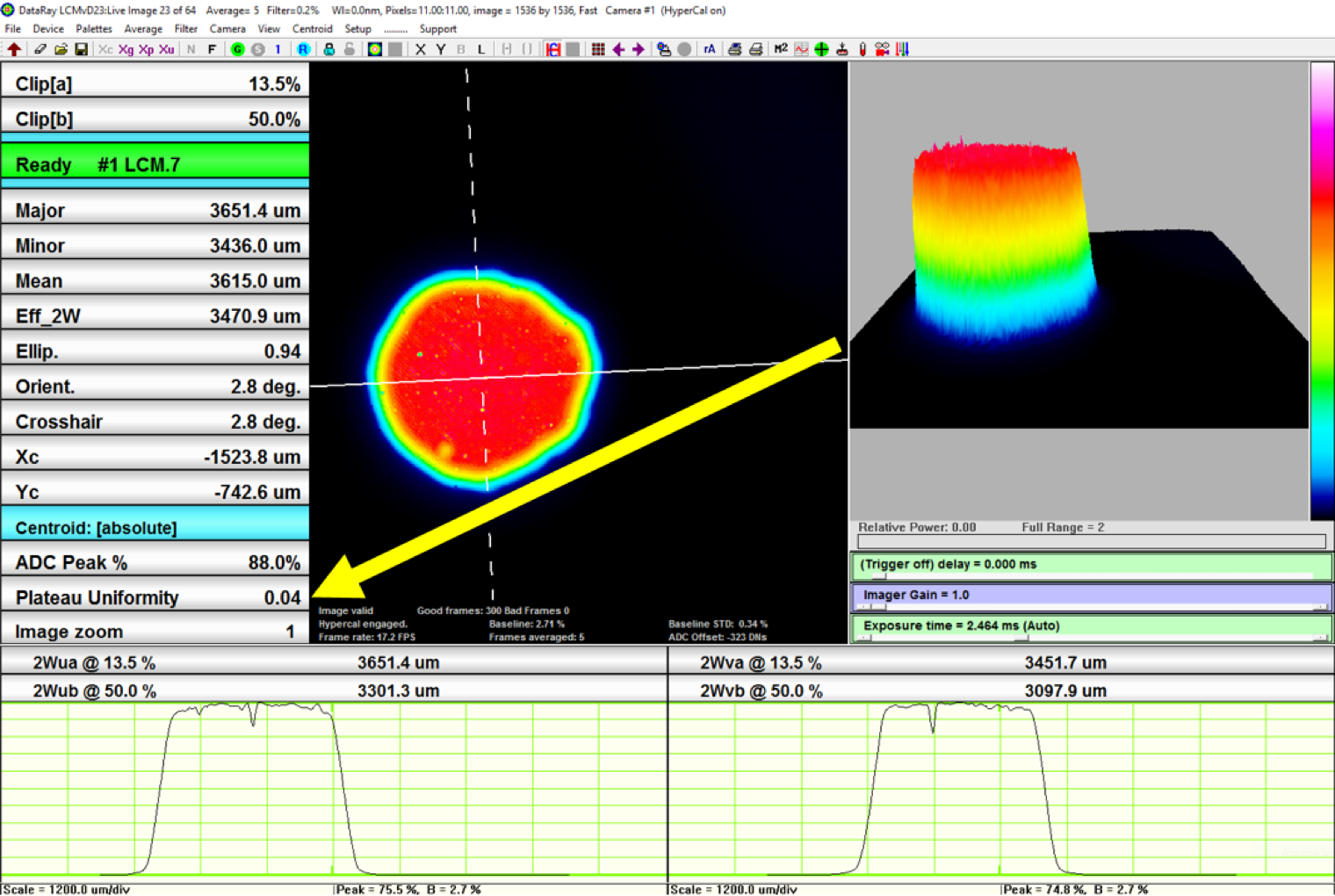Figure 2: Location of plateau uniformity measurement
in DataRay software. The measurement must first be enabled.

Mathematically a histogram (mi) is a function detailing the number of observations that can be placed into a bin of a certain width . Therefore, for a total number of observations n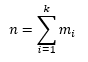Where k is the number of bins. The histogram can be graphically represented by bars. The x-coordinates of each bar are defined by the bins position and width Δx while the y coordinates are defined by the value mi. In our application, the histogram takes the various pixel intensity values across the sensor and puts them into bins representing their intensity.

The number of observations is equal to the number of pixels and henceforth we will discard with the observation notation and refer only to pixels. The x-axis is the range of intensities across the sensor, and the height of the bars is determined by the number of pixels within the bars respective intensity range.

#### Plateau Uniformity

To determine the uniformity of the beam we take the FWHM value for the Gaussian distribution found on the histogram (see Figure 3). The ISO 13694 standard gives the general equation for determining the plateau uniformity for CW lasers asWhere E(x,y,z) is the power density distribution. For pulse lasers, the equation is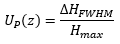With the power density distribution replaced by the energy density distribution H(x,y,z). ΔEFWHMHFWHM) is the FWHM value near the peak Emax (Hmax) on the histogram. As the beam grows closer to the theoretical flat-top, Up (z) → 0. Since the plateau uniformity is a normalized value it follows that 0 < Up (z) < 1. The plateau uniformity measurement is not natively enabled in the DataRay software. To enable the measurement, click Setup in the menu bar. From the Setup menu, click the Enable Plateau Uniformity option. Once enabled, the plateau uniformity value can be found between the ADC Peak % button and the Image Zoom button (see Figure 2).

#### Cutoff

From the histogram of the flat-top beam, we see a large spike that dominates the histogram of the flat-top near 3% of the peak intensity (see Figure 3). This spike derives predominately from the unilluminated pixels on the sensor surface (see Figure 1). To remove the spike and look at meaningful data, only pixels whose values are greater than 13.5% of the peak intensity are used in the histogram (see Figure 3).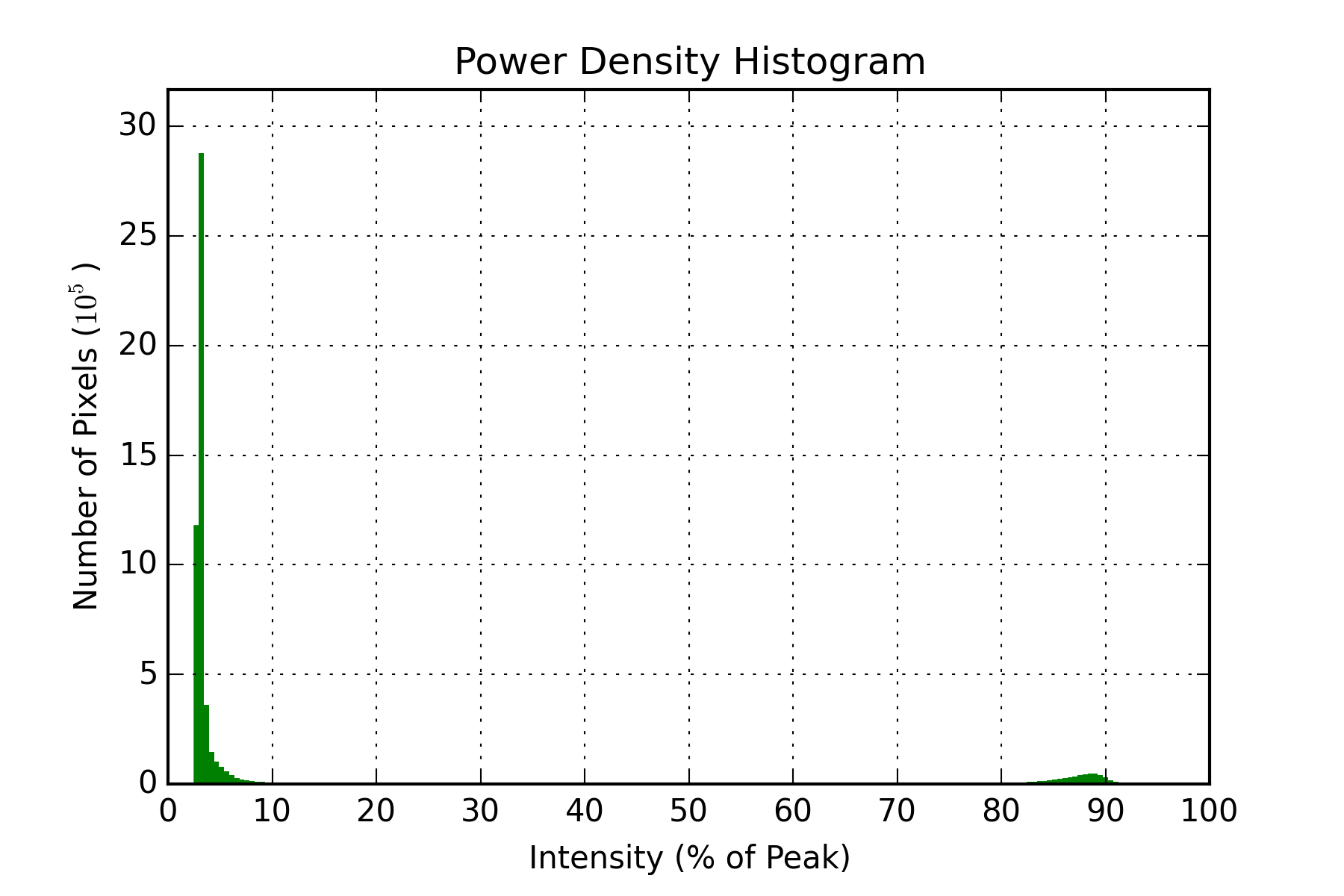Figure 3: Flat-top beam without cutoff (from Figure 1).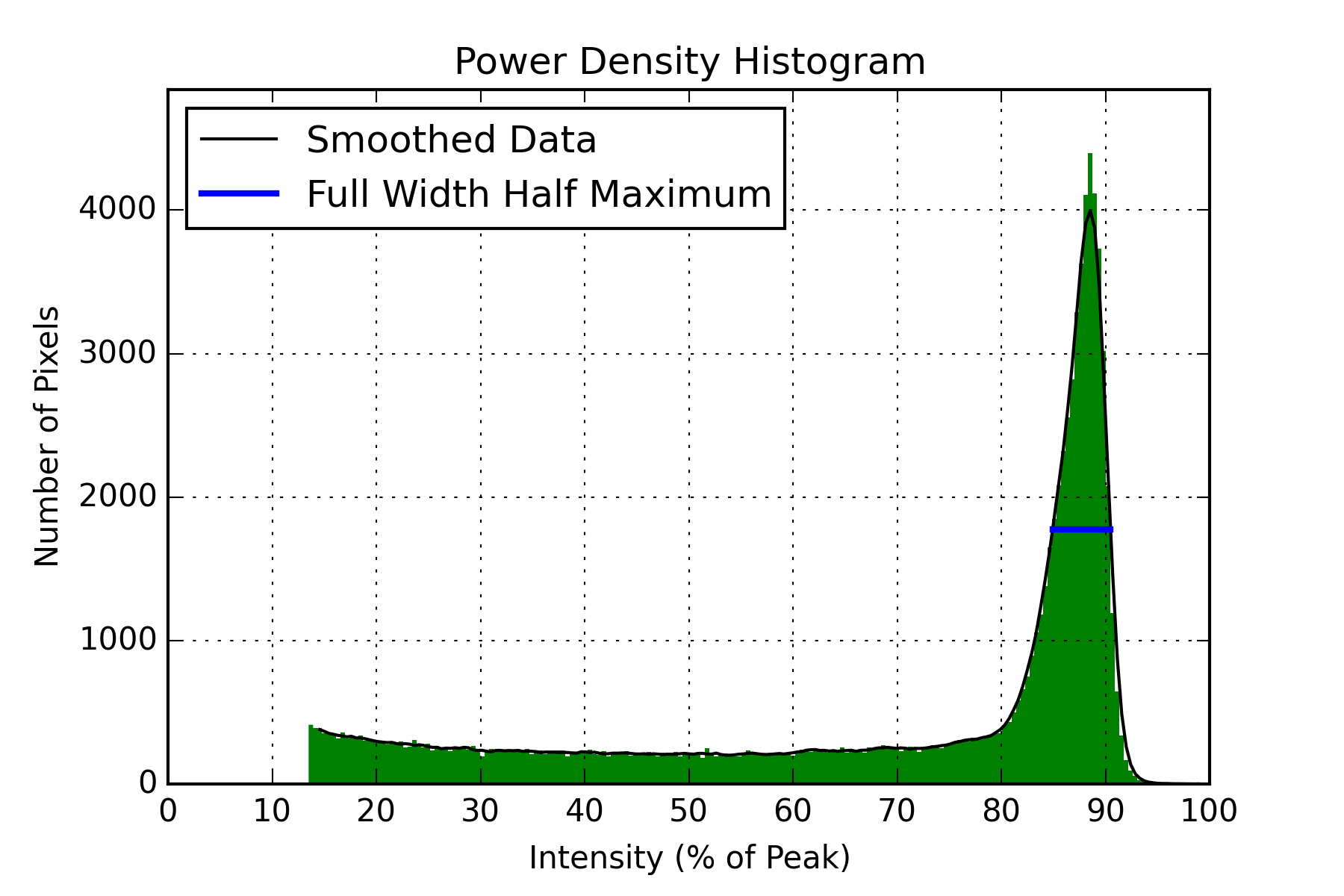Figure 4: Flat-top beam with cutoff (from Figure 1).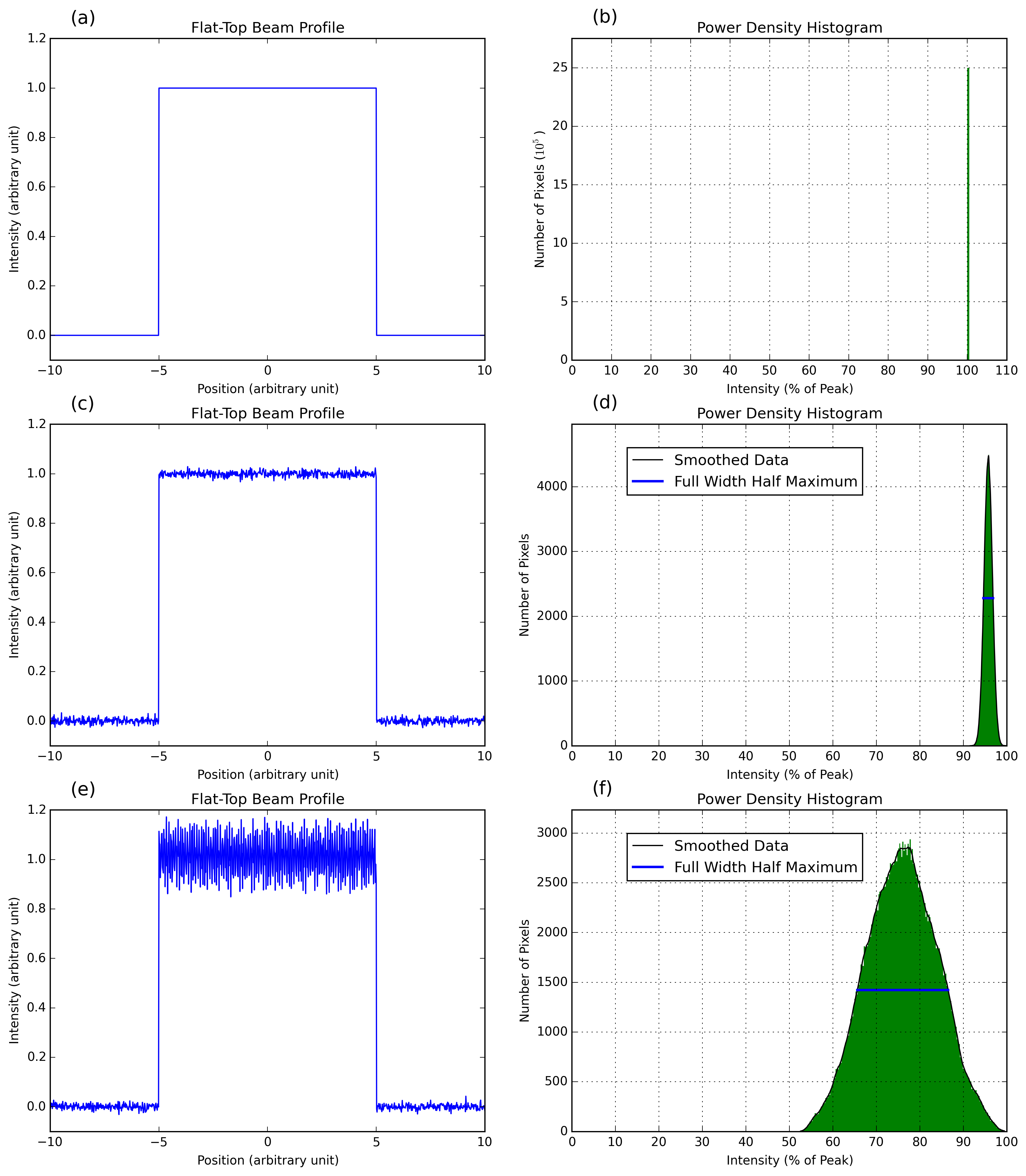Figure 5: (a) Represents a theoretically perfect square flat-top beam. (b) The power density histogram for (a). (c) A generated flat-top beam with Gaussian noise. (d) The power density histogram for (c) with the smoothed data (solid black) after filtering. The Full Width Half Maximum (solid blue) is calculated with DataRay’s proprietary software. (e) The flat-top beam with both noise and 4 different sinusoidal modes in the plateau region. (f) The power density histogram for (e).

#### Theoretical Flat-Top Histograms

For a perfect flat-top beam (see Figure 5a), all the pixels have the same intensity value and are placed in a single bin (see Figure 5b). In this case, the plateau uniformity value is the width of the bin. Since Δx → 0. In reality, flat-top beams are almost never perfect. We generated a flat-top beam with Gaussian noise and its accompanying histogram (see Figure 5c and Figure 5d). The histogram now displays a Gaussian profile rather than the impulse spike. However, noise in the histogram profile can cause problems for determining the FWHM. DataRay software provides a smoothing algorithm (solid black) before looking for the FWHM. After smoothing the histogram data, the FWHM (solid blue) is easily calculated. For the flat-top with Gaussian noise, Up (z) → .022. However, a perfect flat-top beam with Gaussian noise is almost impossible to create. In practice a flat-top beam has different modes in the plateau region. We generated a flat-top beam with several different sinusoidal modes running along both the x and y axes (see Figure 5e). The Gaussian distribution begins to spread in the power density histogram (see Figure 5f) and we see that the Up (z) → .275. This series of generated flat-tops demonstrates the usefulness of the plateau uniformity measurement. The perfect flat-top has Up (z) = 0, the flat-top with Gaussian noise has Up (z) = 0.022, and the flat-top with sinusoidal modes has Up (z) = 0.275. Therefore, as the flat-top deviates from the perfect flat-top, Up (z) → 1.

Although the flat-tops generated show a perfect step when moving to and from a plateau region, with real optics a Gaussian like drop off in intensity is seen at the edges of the plateau (see Figure 1). Therefore, the histogram for a flat-top in real world conditions displays not only a Gaussian distribution, but lower level intensity values that come from the Gaussian component of the beam (see Figure 4). As long as the low level intensity values reside below the half maximum value, they will not affect the FWHM measurement calculation.

#### Application Note

An Application Note related to the topic described about is available for review here.